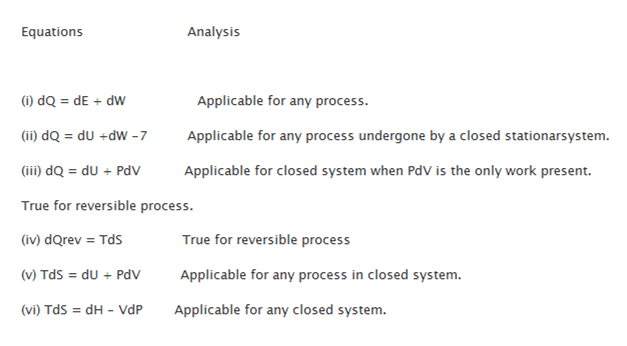Given that Thermodynamics is specifically that branch of physics, which deal with relation between various forms of energy including heat, it is to be noted that certain properties can be measured while others are to be left behind.

While analyzing thermodynamic properties as temperature, volume and pressure, it can be seen that these values are measured. While there are other properties which cannot be measured correctly and hence require thermodynamic relations for determining their features in a correct manner. From these relations, further properties can be determined.

Mathematical Relations: If you wish to define the simple compressible system that has a specific mass and composition, it is necessary that 2 intensive properties of independence are required.

Various Thermodynamic equations are placed as:

Thermodynamics Equations can be given as-